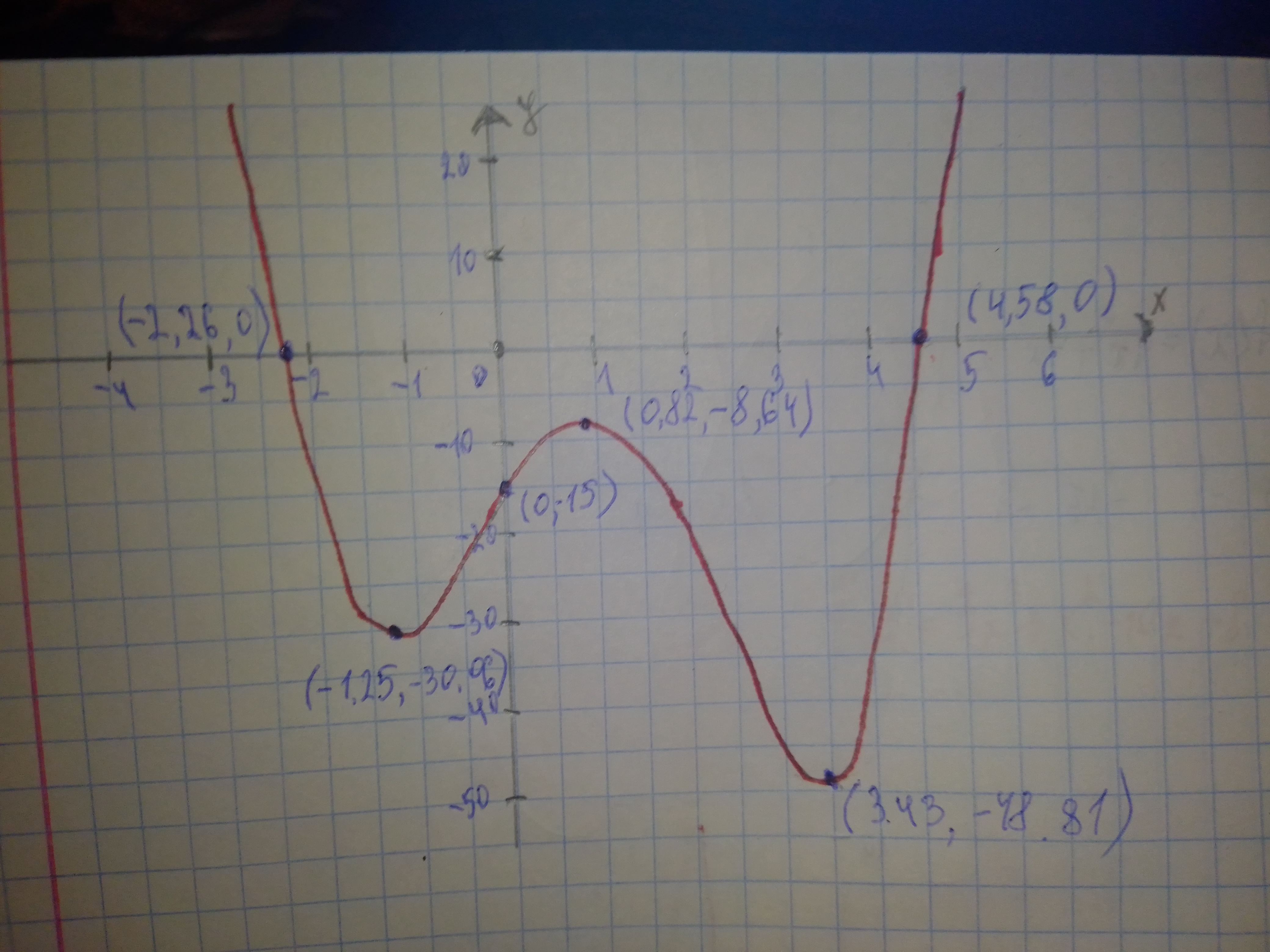Ask question

# Graph each polynomial function. f(x)=x^{4}-4x^{3}-5x^{2}+14x-15# Graph each polynomial function. f(x)=x^{4}-4x^{3}-5x^{2}+14x-15

Question
Polynomial graphsasked 2020-11-23
Graph each polynomial function. $$\displaystyle{f{{\left({x}\right)}}}={x}^{{{4}}}-{4}{x}^{{{3}}}-{5}{x}^{{{2}}}+{14}{x}-{15}$$

## Answers (1)2020-11-24
Use a graphing calculator to graph $$\displaystyle{f{{\left({x}\right)}}}={x}^{{{4}}}-{4}{x}^{{{3}}}-{5}{x}^{{{2}}}+{14}{x}-{15}$$ The x-intercepts ar (-2.26, 0) and (4.58, 0) The y-intercept is (0, -15)### Relevant Questionsasked 2021-01-10
Graph each polynomial function. Factor first if the expression is not in factored form. $$\displaystyle{f{{\left({x}\right)}}}={\left({4}{x}+{3}\right)}{\left({x}+{2}\right)}^{{{2}}}$$asked 2021-02-12
Graph each polynomial function. Factor first if the expression is not in factored form. $$\displaystyle{f{{\left({x}\right)}}}={x}^{{{2}}}{\left({x}-{5}\right)}{\left({x}+{3}\right)}{\left({x}-{1}\right)}$$asked 2020-10-18
Graph each polynomial function. PSKf(x)=x^{4}\ -\ x^{3}\ -\ 6x^{2}\ +\ 4x\ +\ 8 PSZasked 2021-01-22
Graph each polynomial function. Factor first if the expression is not in factored form.
$$\displaystyle{f{{\left({x}\right)}}}={x}^{{{2}}}{\left({x}+{1}\right)}{\left({x}-{1}\right)}$$asked 2021-01-13
Graph each polynomial function. Factor first if the expression is not in factored form.
$$\displaystyle{f{{\left({x}\right)}}}={\left({3}{x}-{1}\right)}{\left({x}+{2}\right)}^{{{2}}}$$asked 2021-02-03

Graph each polynomial function. $$f(x)=2x^{3}\ -\ x^{2}\ +\ 2x\ -\ 1$$asked 2021-02-20
Graph each polynomial function. $$\displaystyle{f{{\left({x}\right)}}}={4}{x}^{{{4}}}+{7}{x}^{{{2}}}-{2}$$asked 2020-12-02
Graph each polynomial function.$$\displaystyle{f{{\left({x}\right)}}}={x}^{{{3}}}+{2}{x}^{{{2}}}-{5}{x}-{6}$$asked 2020-12-30
Graph the polynomial function.
$$\displaystyle{f{{\left({x}\right)}}}={x}^{{{5}}}+{x}$$asked 2021-02-25
a) Identify the parameters a, b, h, and k in the polynomial $$\displaystyle{y}={\frac{{{1}}}{{{3}}}}{\left({x}+{3}\right)}^{{{3}}}-{2}$$ Describe how each parameter transforms the base function $$\displaystyle{y}={x}^{{{3}}}$$.
b) State the domain and range of the transformed function.
c) Sketch graphs of the base function and the transformed function on the same set of axes.
...